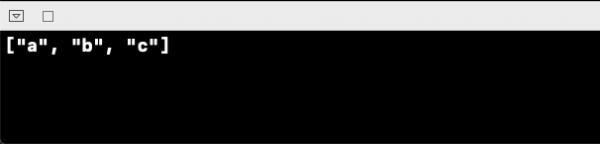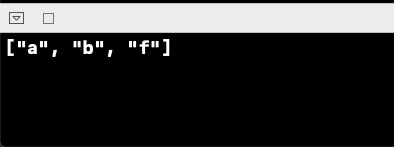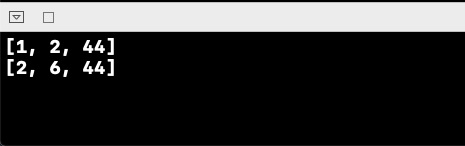# How to remove Specific Element from a Swift Array?

To remove a specific object from an element in swift, we can use multiple ways of doing it. Let’s see this in the playground with help of an example.

First, let’s create an array of String.

var arrayOfString = ["a","b","c","f"]

We’ll do it with the following methods as shown below:

## Method 1 − Using the filter method of the array.

Arrays in swift have a filter method, which filters the array object depending on some conditions and returns an array of new objects.

let modifiedArray = arrayOfString.filter { $0 != "f" } print(modifiedArray) When we run the above code, we get the following result.## Method 2 − Removing using the indexPath Now, we’ll use the indexPath of the object to remove it from the array. if arrayOfString.contains("c") { let index = arrayOfString.firstIndex(of: "c") arrayOfString.remove(at: index!) print(arrayOfString) } When we run the above code, we get the following result.Let’s see one more example of both methods, with numbers. var arry = [1,2,6,44] let modifiedArray = arry.filter {$0 != 6 }
print(modifiedArray)
if arry.contains(1) {
let index = arry.firstIndex(of: 1)
arry.remove(at: index!)
print(arry)
}

We get the following output when we run the above code.